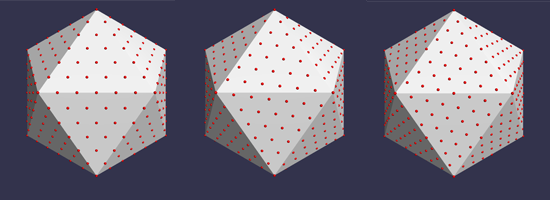# Creating Polyhedra Shapes

## Polyhedra

There are a multitude of polyhedra, too many for all to be created by individual names. Often their names take too long anyway and Babylon.js provides 15 common ones via number. There are many beyond these 15 and those can be created from custom data sets provided in the form of Playground examples.

The twenty sided icosahedron has the interesting property of approximating a sphere. By dividing each face into triangles and then mapping the resulting vertices onto a sphere it gives a better approximation of a sphere. The solids formed are called icospheres and provide a more regular distribution of triangles around a sphere then the CreateSphere method.

Other sphere approximations can be provided by geodesic and Goldberg polyhedra. Like the icosphere a geodesic polyhedron is based on an icosahedron and formed from equilateral triangles, in this case, however, their arrangement depends on two parameters, m and n, both integers. A Goldberg polyhedron is the dual of a geodesic one, and vice-versa. A dual of a polyhedron is formed by mapping its vertices to the faces of the dual and its faces to the dual's vertices. A Goldberg polyhedron is made up of 12 pentagonal and many hexagonal faces and has the advantage of a planet shaped hexagon-grid world.The Arrangement of Vertices for a Geodesic Poly with m = 6 and n = 0, 1, 2 in order